## Bayes Theorem

Probabilty that A occurs given B has occured P(A | B)

The Bayes Theorem is a method of finding what the proababilty is of something ocuuring (A) given that something else has just occured (B).

## Example for Bayes Theorem

Two machines produce wrenches. We know that:

• Machine 1 : Produces 30 Wrenches Per Hour
• Machine 2 : Produces 20 Wrenches Per Hour
• 1% of all wrenches are defective, where 50% of the wrenches that are defective are from machine 1 and 50% from machine 2.

Question: What is the probabilty that a part produced by machine 2 is defective?

• P M1) = 30/50 = 0.6
• P (M2) = 20/50 = 0.4
• P (D) = 0.01
• P (M1 | D) = 0.5 and P(M2 | D) = 0.5
• P (D | M2) = ??? (Question statement)

## Naive Bayes Classifier

The implementation for a NAive Bayes theorem will be a supervised machine learning algorithm used to classify data with previous known classes.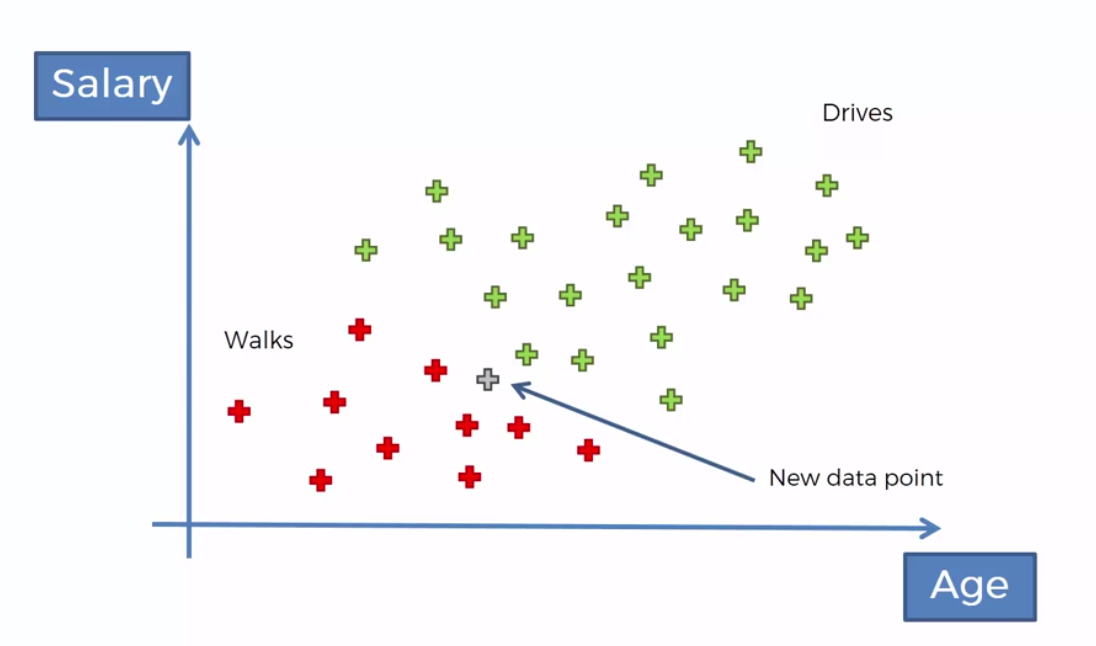How it Works:

1. Apply Bayes Theorem in this example we’ll use it to find the proabalilty that this person walks based on his features (the specific age and salary of that data point). Calculate the probability of each of the components of BAyes Theorem.
2. Apply Theorem again, in this case it would be to find the probability that the new dataset Drives based on its features (age and salary).
3. Compare the two and assign a class to the dataset.

### Following the Example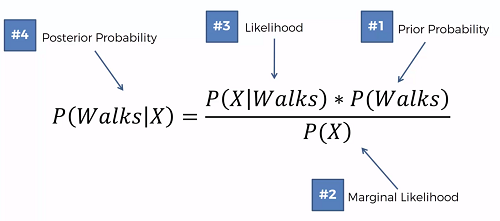1. Bayes Theorem Step 1

• Prior Probabilty Calculation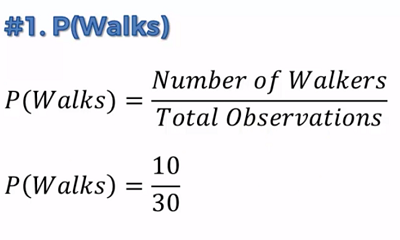• Marginal Likehood : Select a radius around the data point. P(X) is the probabilty of any given point to fall within that selected radius.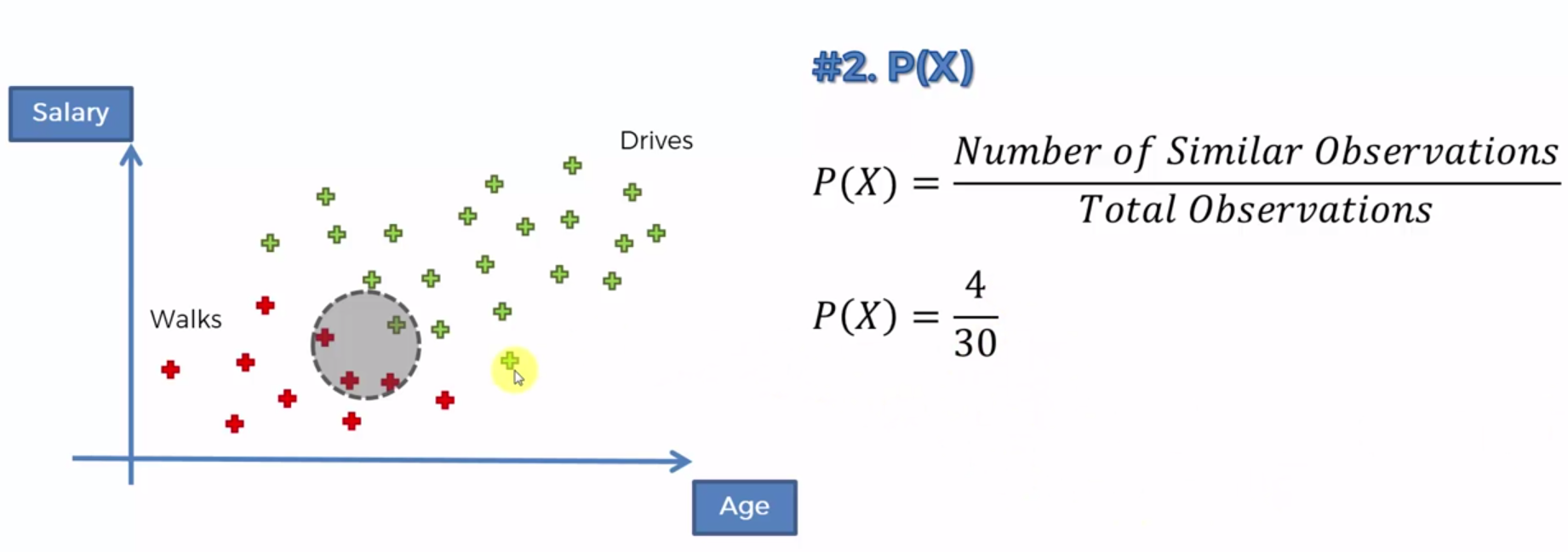• Likelihood: Same radius. P(X | Walks) : Probability of the data point would be in this circle given that that datapoint walks.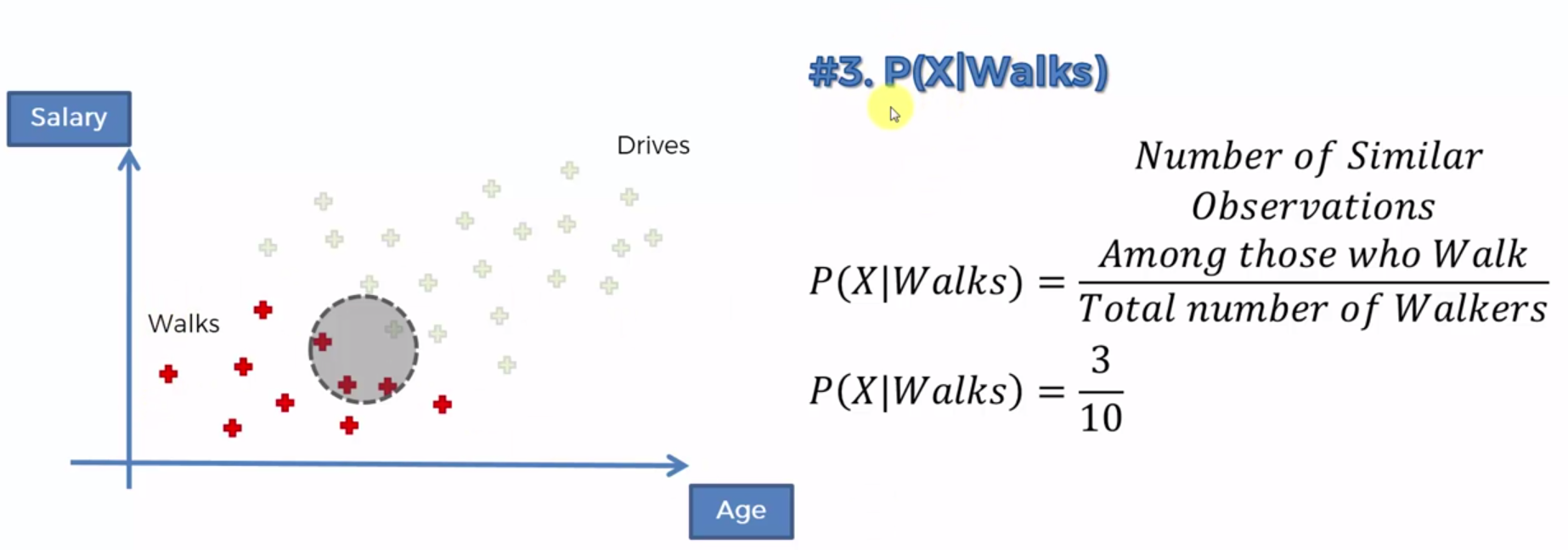• Calculate Bayes Theorem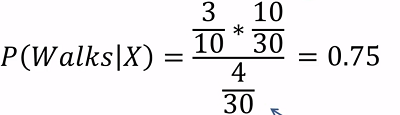2. Bayes Theorem Step 2 (repeat)3. Since in this case the probability of the data point walking is greater than driving we can say that the data point is assigned to walking

## Naive Bayes Theorem in Python

Use the classification template to set everything up.

Create the classifier and set it up

from sklearn.naive_bayes import GaussianNB
classifier = GaussianNB()
classifier.fit(X_train, y_train)


run code…